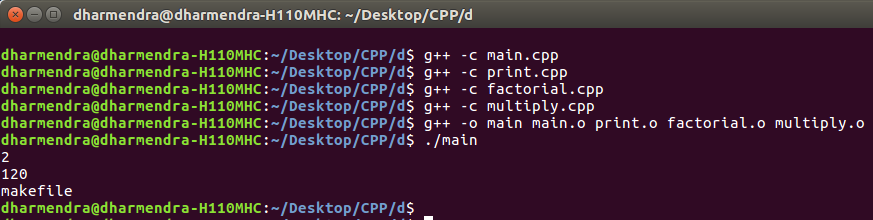Open in App
Not now

# MakeFile in C++ and its applications

• Difficulty Level : Easy
• Last Updated : 09 Sep, 2022

Given a C++ program and the task is to break the entire program in the form of Makefile. It is basically used to create .cpp file and .h file for each class/functions and its functionalities and later link them all through a Makefile command available in C++. Advantages:

• It makes codes more concise and clear to read and debug.
• No need to compile entire program every time whenever you make a change to a functionality or a class. Makefile will automatically compile only those files where change has occurred.
• Generally, in long codes or projects, Makefile is widely used in order to present project in more systematic and efficient way.

Example: Create a program to find the factorial and multiplication of numbers and print it. Traditional Way

## CPP

 `// Program to calculate factorial and``// multiplication of two numbers.``#include``using` `namespace` `std;` `// Function to find factorial``int` `factorial(``int` `n)``{``    ``if` `(n == 1)``        ``return` `1;``    ``// Recursive Function to find``    ``// factorial``    ``return` `n * factorial(n - 1);``}` `// Function to multiply two numbers``int` `multiply(``int` `a, ``int` `b)``{``    ``return` `a * b;``}` `// Function to print``void` `print()``{``    ``cout << "makefile" << endl;``}` `// Driver code``int` `main()``{``    ``int` `a = 1;``    ``int` `b = 2;``    ``cout << multiply(a, b) << endl;``    ``int` `fact = 5;``    ``cout << factorial(5) << endl;``    ``print();``    ``return` `0;``}`

Output:

```2
120
makefile```

Use Makefile to run the above program:

• File Name: main.cpp

## CPP

 `#include ` `// Note function.h which has all functions``// definations has been included``#include "function.h"` `using` `namespace` `std;` `// Main program``int` `main()``{``    ``int` `num1 = 1;``    ``int` `num2 = 2;``    ``cout << multiply(num1, num2) << endl;``    ``int` `num3 = 5;``    ``cout << factorial(num3) << endl;``    ``print();``}`

• File Name: print.cpp

## CPP

 `#include ` `// Definition of print function is``// present in function.h file``#include "function.h"``using` `namespace` `std;` `void` `print()``{``    ``cout < "makefile" << endl;``}`

• File Name: factorial.cpp

## CPP

 `#include ` `// Definition of factorial function``// is present in function.h file``#include "function.h"``using` `namespace` `std;` `// Recursive factorial program``int` `factorial(``int` `n)``{``    ``if` `(n == 1)``        ``return` `1;``    ``return` `n * factorial(n - 1);``}`

• File Name: multiply.cpp

## CPP

 `#include ` `// Definition of multiply function``// is present in function.h file``#include "function.h"``using` `namespace` `std;` `int` `multiply(``int` `a, ``int` `b)``{``    ``return` `a * b;``}`

• File Name: functions.h

## CPP

 `#ifndef FUNCTIONS_H``#define FUNCTIONS_H` `void` `print();``int` `factorial(``int``);``int` `multiply(``int``, ``int``);` `#endif`

Commands to Compile and Run above program:

```Open Terminal and type commands:
g++ -c main.cpp
g++ -c print.cpp
g++ -c factorial.cpp
g++ -c multiply.cpp
g++ -o main.o print.o factorial.o multiply.o
./main

Note: g++ -c filename.cpp is used to create object file.```My Personal Notes arrow_drop_up